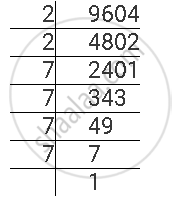Share

# Find the Square Roots of the Given Numbers by the Prime Factorisation Method. 9604 - Mathematics

Course

#### Question

Find the square roots of the given numbers by the Prime Factorisation Method.

9604

#### Solution

9604 can be factorised as follows.9604 = 2 × 2 × 7 × 7 × 7 × 7

∴ sqrt(9604) = 2 x 7 x 7 = 98

Is there an error in this question or solution?